The plot_outlier() visualize outlier information for diagnosing the quality of the numerical data.

plot_outlier(.data, ...)

# S3 method for data.frame
plot_outlier(
.data,
...,
col = "steelblue",
typographic = TRUE,
base_family = NULL
)

## Arguments

.data a data.frame or a tbl_df. one or more unquoted expressions separated by commas. You can treat variable names like they are positions. Positive values select variables; negative values to drop variables. If the first expression is negative, plot_outlier() will automatically start with all variables. These arguments are automatically quoted and evaluated in a context where column names represent column positions. They support unquoting and splicing. a color to be used to fill the bars. The default is "steelblue". logical. Whether to apply focuses on typographic elements to ggplot2 visualization. character. The name of the base font family to use for the visualization. If not specified, the font defined in dlookr is applied. (See details) The default is TRUE. if TRUE provides a base theme that focuses on typographic elements using hrbrthemes package.

## Details

The scope of the diagnosis is the provide a outlier information. Since the plot is drawn for each variable, if you specify more than one variable in the ... argument, the specified number of plots are drawn.

The base_family is selected from "Roboto Condensed", "Liberation Sans Narrow", "NanumSquare", "Noto Sans Korean". If you want to use a different font, use it after loading the Google font with import_google_font().

## Outlier diagnostic information

The plot derived from the numerical data diagnosis is as follows.

• With outliers box plot

• Without outliers box plot

• With outliers histogram

• Without outliers histogram

See vignette("diagonosis") for an introduction to these concepts.

plot_outlier.tbl_dbi, diagnose_outlier.data.frame.

## Examples

# \donttest{
# Visualization of all numerical variables
plot_outlier(heartfailure)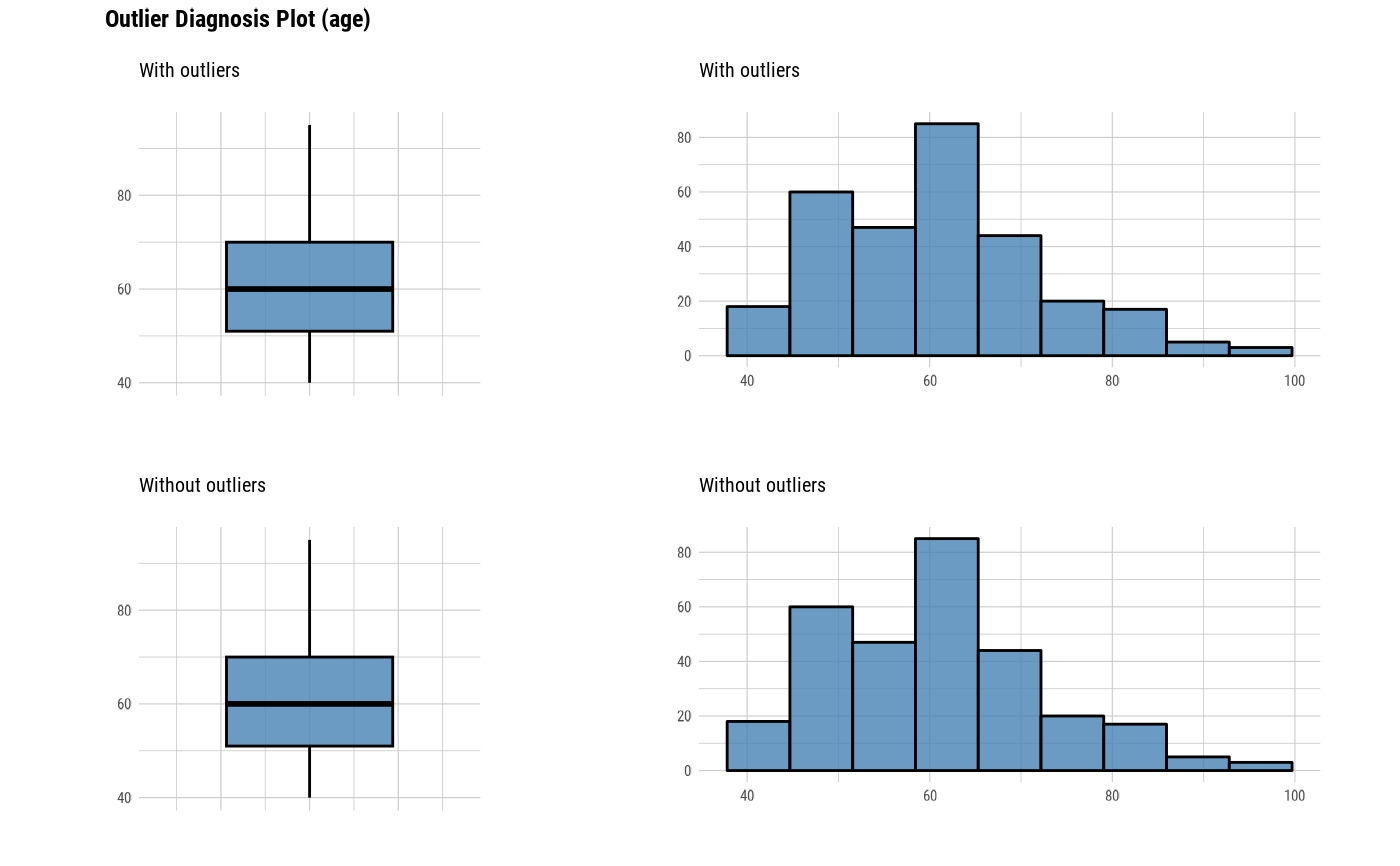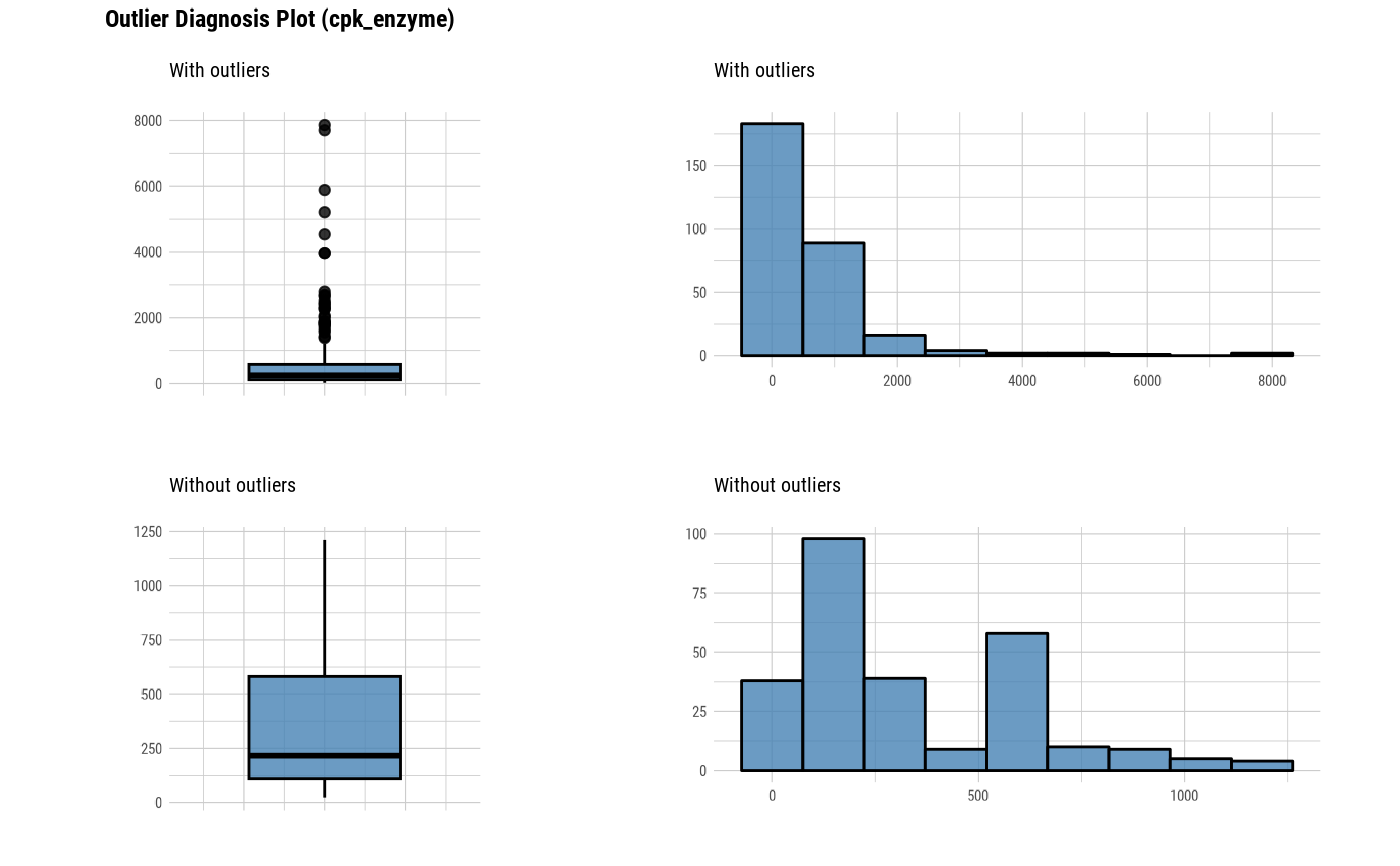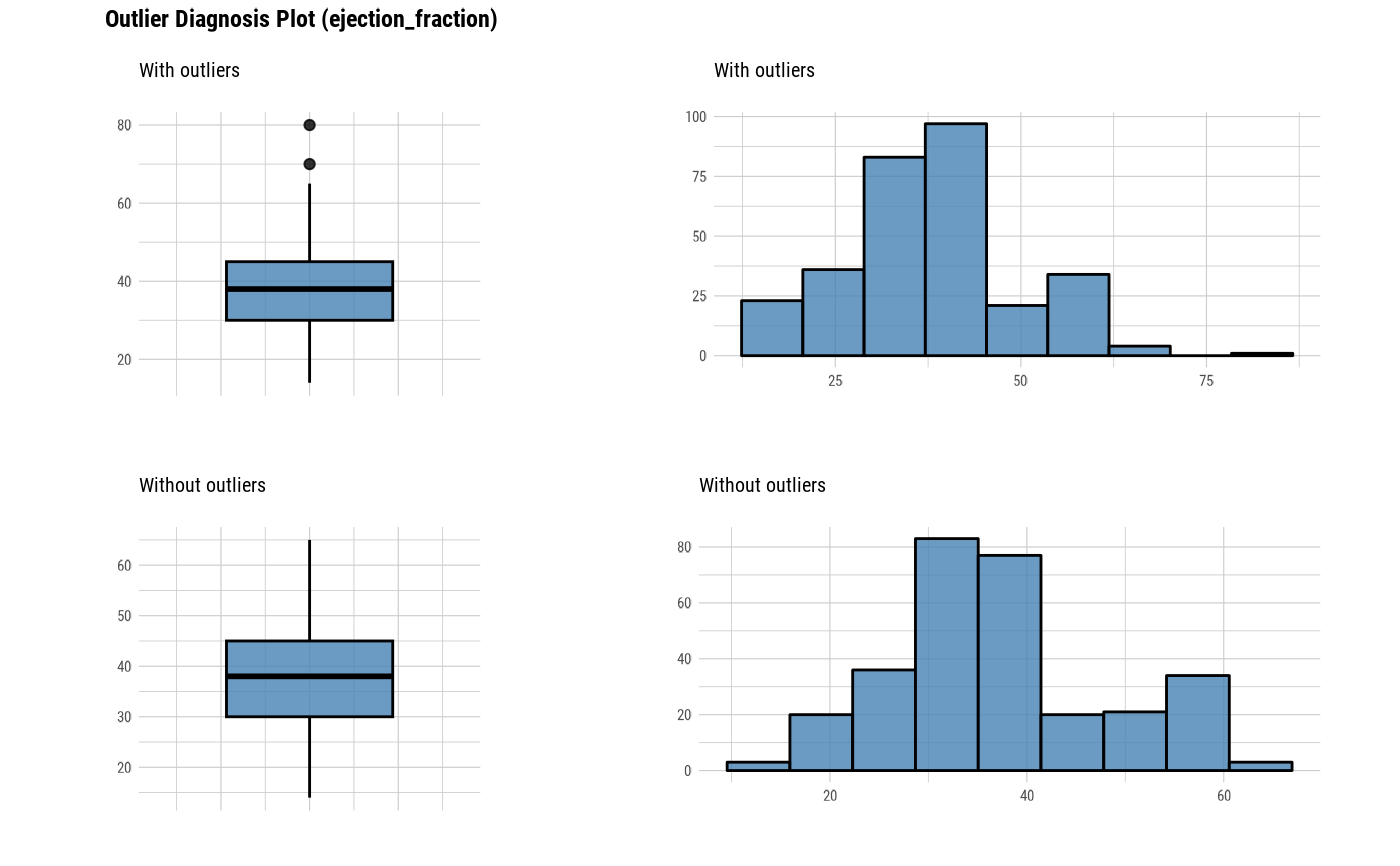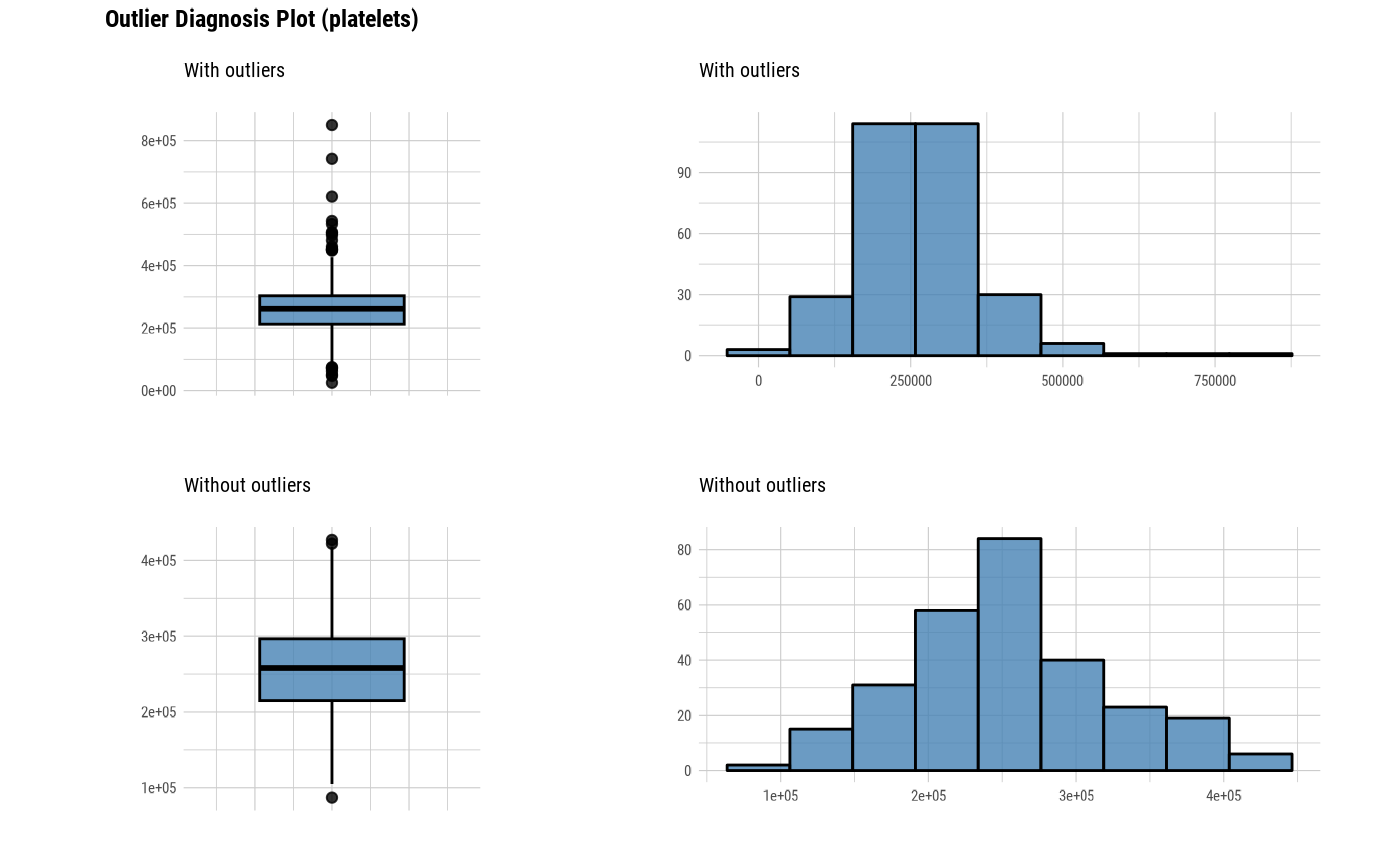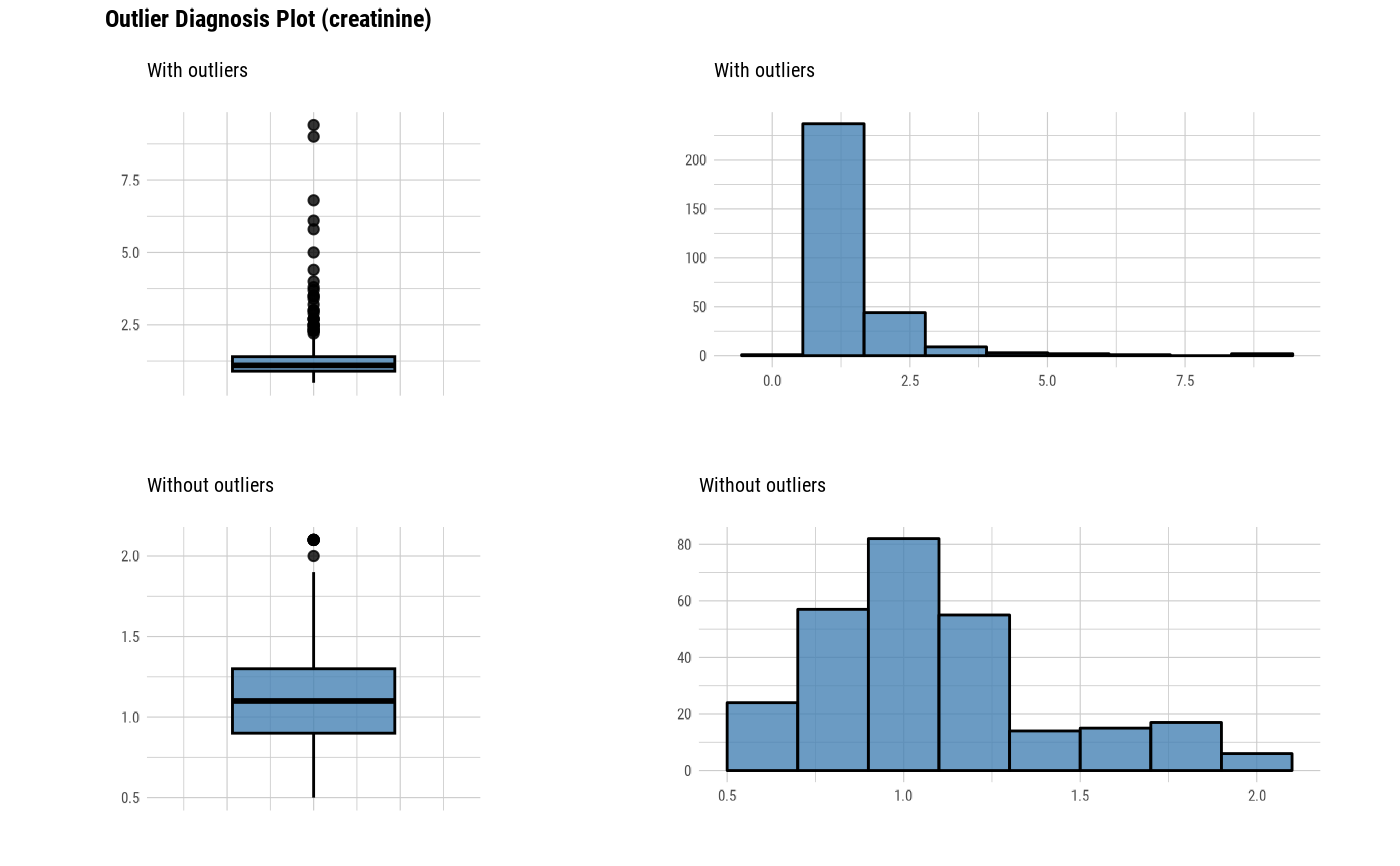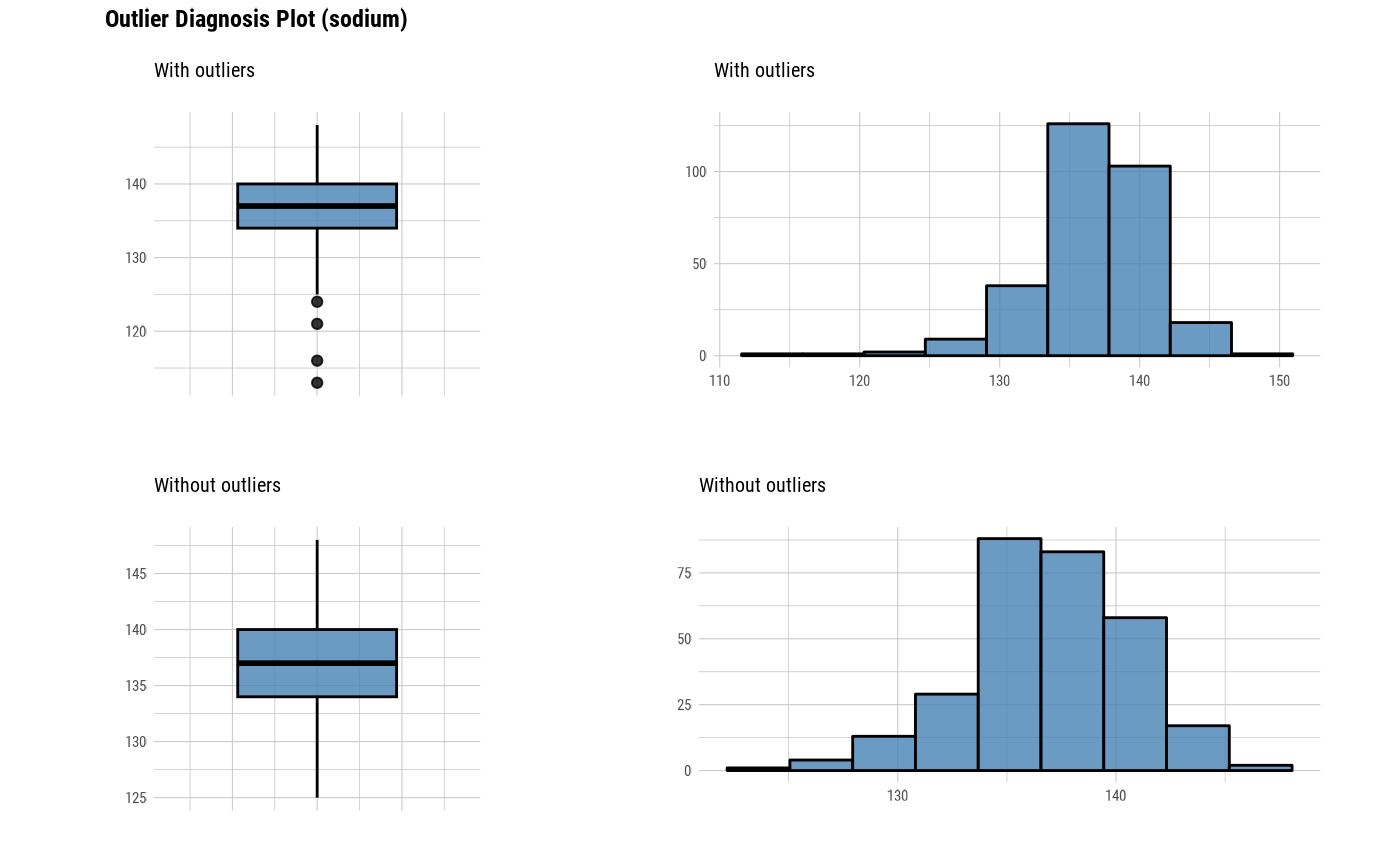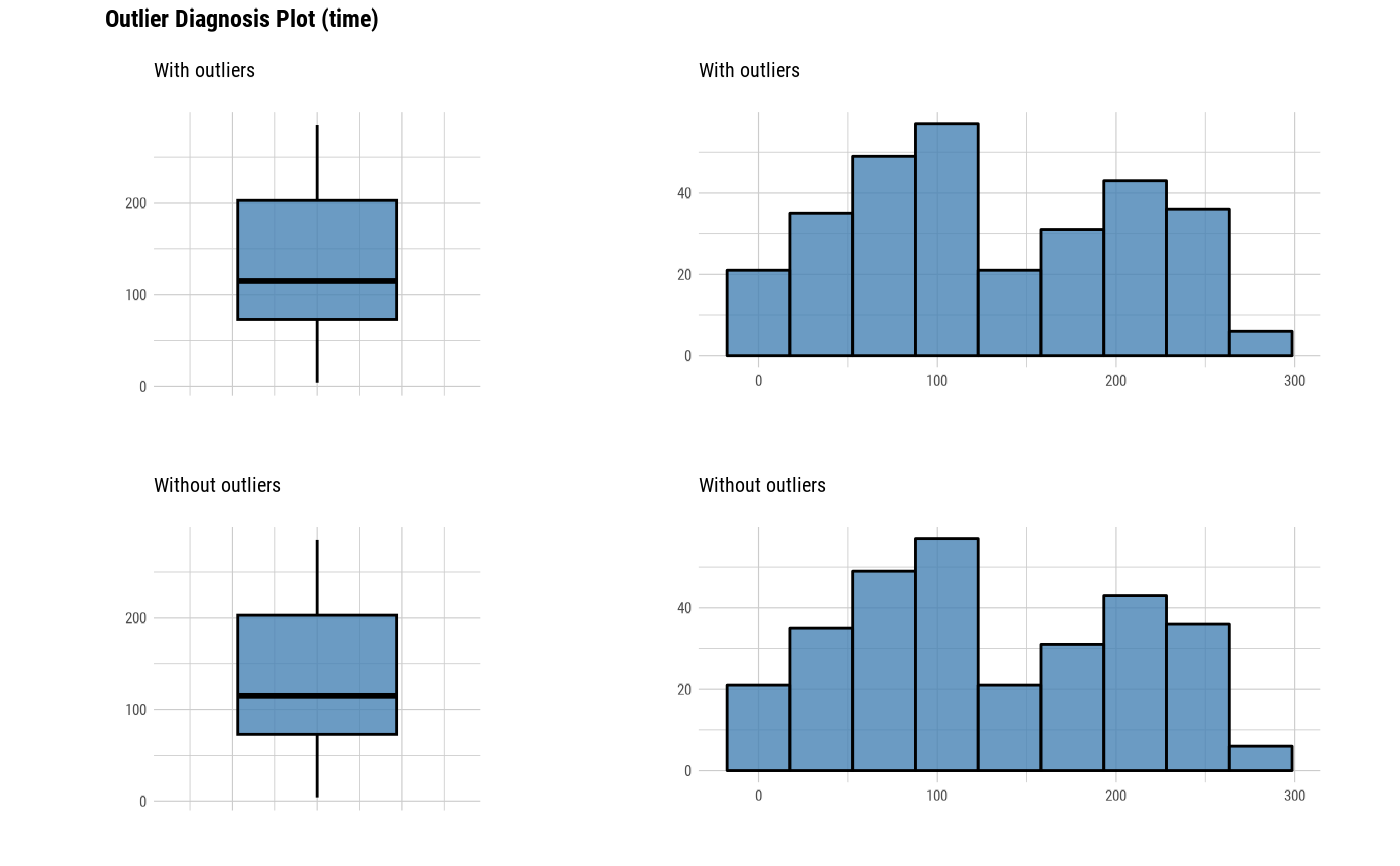# Select the variable to diagnose
plot_outlier(heartfailure, cpk_enzyme, sodium)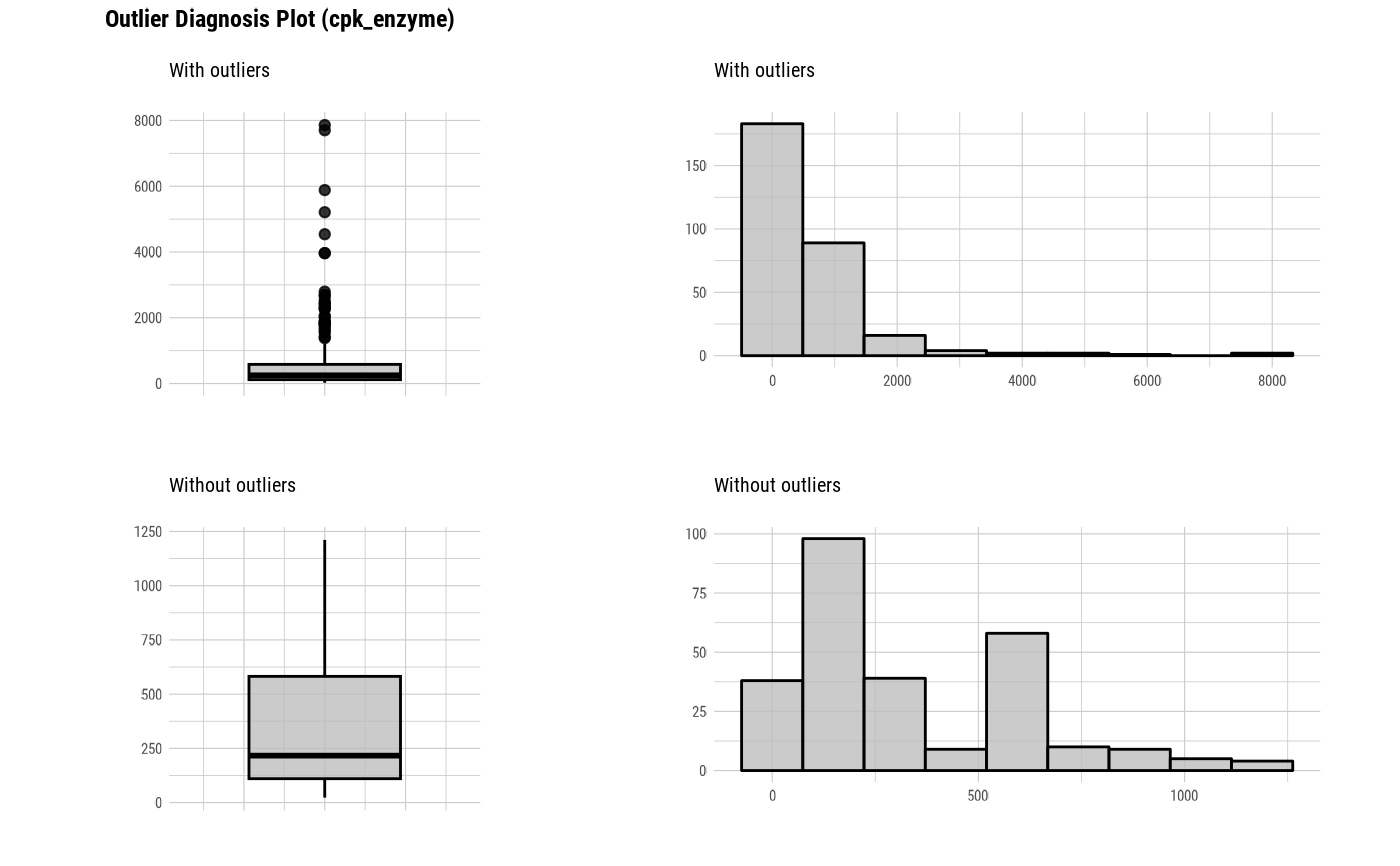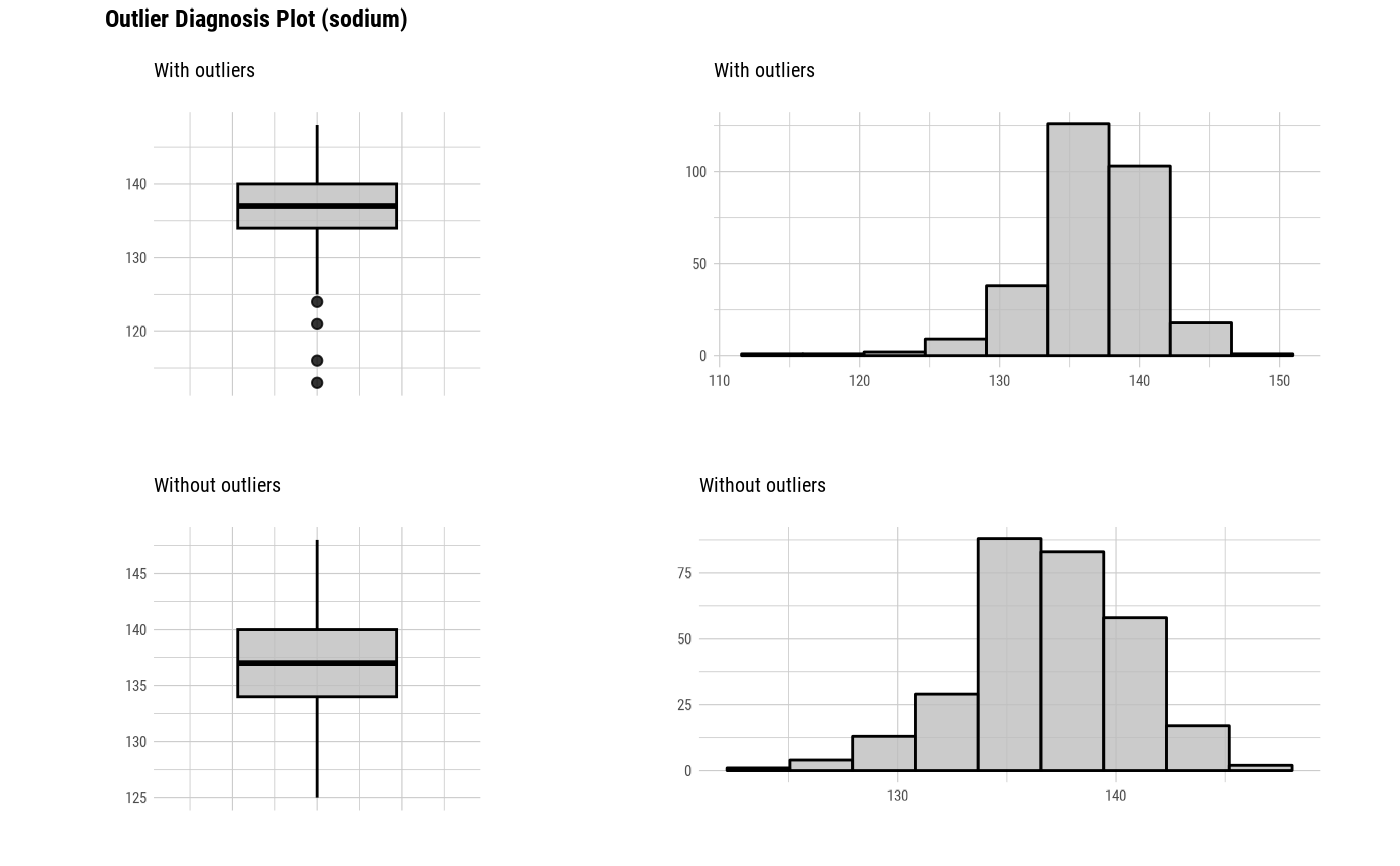plot_outlier(heartfailure, -cpk_enzyme, -sodium)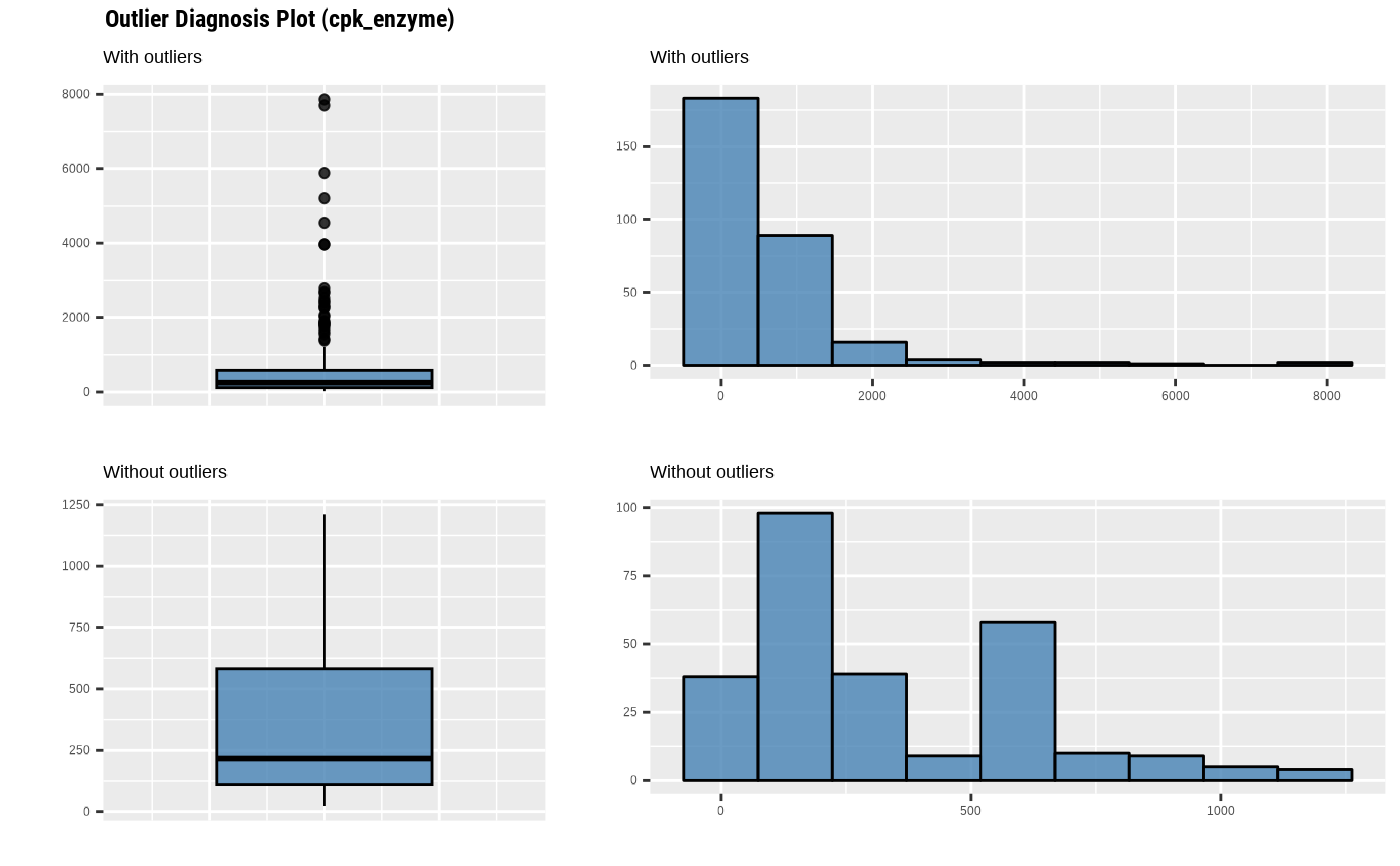plot_outlier(heartfailure, "cpk_enzyme", "sodium")plot_outlier(heartfailure, 7)# Using the col argument
plot_outlier(heartfailure, cpk_enzyme, col = "gray")# Not allow typographic argument
plot_outlier(heartfailure, cpk_enzyme, typographic = FALSE)# Using pipes ---------------------------------
library(dplyr)

# Visualization of all numerical variables
heartfailure %>%
plot_outlier()# Positive values select variables
heartfailure %>%
plot_outlier(cpk_enzyme, sodium)# Negative values to drop variables
heartfailure %>%
plot_outlier(-cpk_enzyme, -sodium)# Positions values select variables
heartfailure %>%
plot_outlier(7)# Negative values to drop variables
heartfailure %>%
plot_outlier(-1, -5)# Using pipes & dplyr -------------------------
# Visualization of numerical variables with a ratio of
# outliers greater than 5%
heartfailure %>%
plot_outlier(heartfailure %>%
diagnose_outlier() %>%
filter(outliers_ratio > 5) %>%
select(variables) %>%
pull())# }